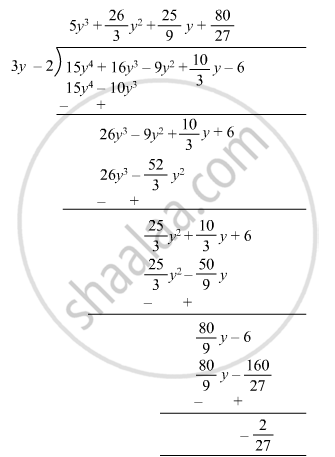Advertisement Remove all ads

# Divide 15y4 + 16y3 + 10 3 Y − 9y2 − 6 by 3y − 2. Write Down the Coefficients of the Terms in the Quotient. - Mathematics

Sum

Divide 15y4 + 16y3 +$\frac{10}{3}$y − 9y2 − 6 by 3y − 2. Write down the coefficients of the terms in the quotient.

Advertisement Remove all ads

#### Solution$\therefore$ Quotient = 5y3 + (26/3)y2 + (25/9)y + (80/27)

Remainder = (-  2/27)

Coefficient of y3 = 5
Coefficient of y2 = (26/3)
Coefficient of y = (25/9)
Constant = (80/27)

Is there an error in this question or solution?
Advertisement Remove all ads

#### APPEARS IN

RD Sharma Class 8 Maths
Chapter 8 Division of Algebraic Expressions
Exercise 8.4 | Q 22 | Page 12
Advertisement Remove all ads

#### Video TutorialsVIEW ALL 

Advertisement Remove all ads
Share
Notifications

View all notifications

Forgot password?
Course